# NCERT Exemplar solutions for Class 11 Physics chapter 6 - Work, Energy and Power [Latest edition]

#### Chapters## Solutions for Chapter 6: Work, Energy and Power

Below listed, you can find solutions for Chapter 6 of CBSE NCERT Exemplar for Class 11 Physics.

Exercises
Exercises [Pages 38 - 49]

### NCERT Exemplar solutions for Class 11 Physics Chapter 6 Work, Energy and Power Exercises [Pages 38 - 49]

#### MCQ I

Exercises | Q 6.1 | Page 38

An electron and a proton are moving under the influence of mutual forces. In calculating the change in the kinetic energy of the system during motion, one ignores the magnetic force of one on another. This is because ______.

• the two magnetic forces are equal and opposite, so they produce no net effect.

• the magnetic forces do no work on each particle.

• the magnetic forces do equal and opposite (but non-zero) work on each particle.

• the magenetic forces are necessarily negligible.

Exercises | Q 6.2 | Page 38

A proton is kept at rest. A positively charged particle is released from rest at a distance d in its field. Consider two experiments; one in which the charged particle is also a proton and in another, a positron. In the same time t, the work done on the two moving charged particles is ______.

• same as the same force law is involved in the two experiments.

• less for the case of a positron, as the positron moves away more rapidly and the force on it weakens.

• more for the case of a positron, as the positron moves away a larger distance.

• same as the work done by charged particle on the stationary proton.

Exercises | Q 6.3 | Page 39

A man squatting on the ground gets straight up and stand. The force of reaction of ground on the man during the process is ______.

• constant and equal to mg in magnitude.

• constant and greater than mg in magnitude.

• variable but always greater than mg.

• at first greater than mg, and later becomes equal to mg.

Exercises | Q 6.4 | Page 39

A bicyclist comes to a skidding stop in 10 m. During this process, the force on the bicycle due to the road is 200 N and is directly opposed to the motion. The work done by the cycle on the road is ______.

• + 2000 J

• – 200 J

• zero

• – 20,000 J

Exercises | Q 6.5 | Page 39

A body is falling freely under the action of gravity alone in vacuum. Which of the following quantities remain constant during the fall?

• Kinetic energy.

• Potential energy.

• Total mechanical energy.

• Total linear momentum.

Exercises | Q 6.6 | Page 39

During inelastic collision between two bodies, which of the following quantities always remain conserved?

• Total kinetic energy.

• Total mechanical energy.

• Total linear momentum.

• Speed of each body.

Exercises | Q 6.7 | Page 39

Two inclined frictionless tracks, one gradual and the other steep meet at A from where two stones are allowed to slide down from rest, one on each track as shown in figure.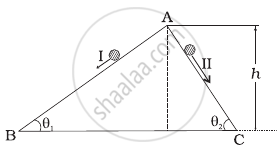Which of the following statement is correct?

• Both the stones reach the bottom at the same time but not with the same speed.

• Both the stones reach the bottom with the same speed and stone I reaches the bottom earlier than stone II.

• Both the stones reach the bottom with the same speed and stone II reaches the bottom earlier than stone I.

• Both the stones reach the bottom at different times and with different speeds.

Exercises | Q 6.8 | Page 40

The potential energy function for a particle executing linear SHM is given by V(x) = 1/2 kx^2 where k is the force constant of the oscillator (Figure). For k = 0.5 N/m, the graph of V(x) versus x is shown in the figure. A particle of total energy E turns back when it reaches x = ±x_m. If V and K indicate the P.E. and K.E., respectively of the particle at x = +x_m, then which of the following is correct?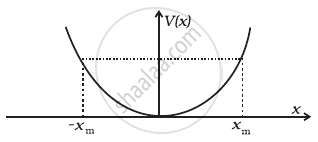• V = O, K = E

• V = E, K = O

• V < E, K = O

• V = O, K < E

Exercises | Q 6.9 | Page 40

Two identical ball bearings in contact with each other and resting on a frictionless table are hit head-on by another ball bearing of the same mass moving initially with a speed V as shown in figure.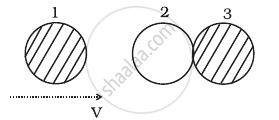If the collision is elastic, which of the following (Figure) is a possible result after collision?

•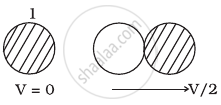•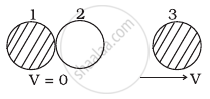•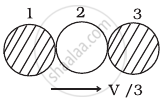•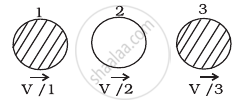Exercises | Q 6.10 | Page 41

A body of mass 0.5 kg travels in a straight line with velocity v = a x3/2 where a = 5 m–1/2s–1. The work done by the net force during its displacement from x = 0 to x = 2 m is ______.

• 1.5 J

• 50 J

• 10 J

• 100 J

Exercises | Q 6.11 | Page 41

A body is moving unidirectionally under the influence of a source of constant power supplying energy. Which of the diagrams shown in figure correctly shows the displacement-time curve for its motion?

•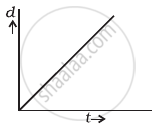•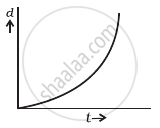•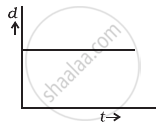•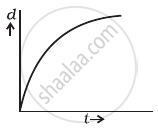Exercises | Q 6.12 | Page 41

Which of the diagrams shown in figure most closely shows the variation in kinetic energy of the earth as it moves once around the sun in its elliptical orbit?

•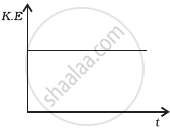•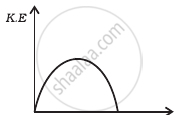•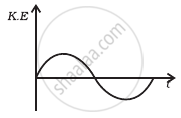•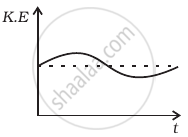Exercises | Q 6.13 | Page 42

Which of the diagrams shown in figure represents variation of total mechanical energy of a pendulum oscillating in air as function of time?

•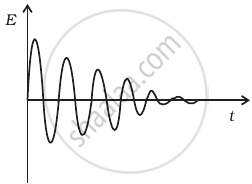•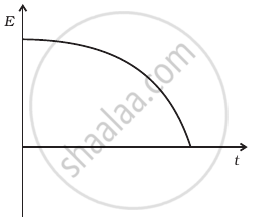•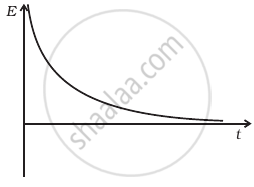•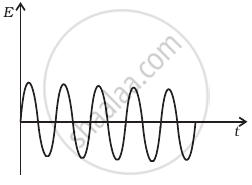Exercises | Q 6.14 | Page 42

A mass of 5 kg is moving along a circular path of radius 1 m. If the mass moves with 300 revolutions per minute, its kinetic energy would be ______.

• 250 π2

• 100 π2

• 5 π2

• 0

Exercises | Q 6.15 | Page 42

A raindrop falling from a height h above ground, attains a near terminal velocity when it has fallen through a height (3/4)h. Which of the diagrams shown in figure correctly shows the change in kinetic and potential energy of the drop during its fall up to the ground?

•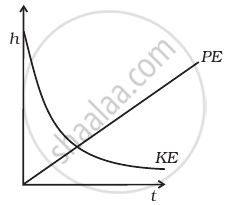•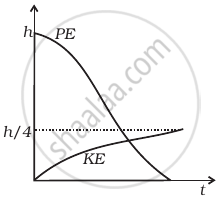•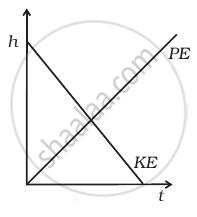•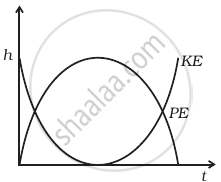Exercises | Q 6.16 | Page 43

In a shotput event an athlete throws the shotput of mass 10 kg with an initial speed of 1 ms–1 at 45° from a height 1.5 m above ground. Assuming air resistance to be negligible and acceleration due to gravity to be 10 ms–2, the kinetic energy of the shotput when it just reaches the ground will be ______.

• 2.5 J

• 5.0 J

• 52.5 J

• 155.0 J

Exercises | Q 6.17 | Page 43

Which of the diagrams in figure correctly shows the change in kinetic energy of an iron sphere falling freely in a lake having sufficient depth to impart it a terminal velocity?

•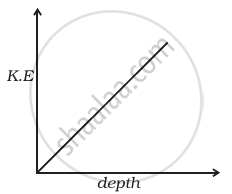•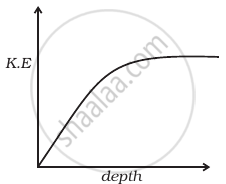•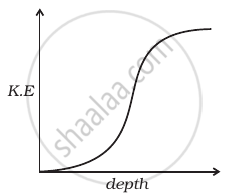•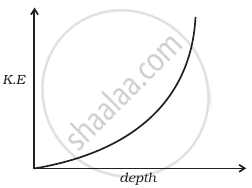Exercises | Q 6.18 | Page 44

A cricket ball of mass 150 g moving with a speed of 126 km/h hits at the middle of the bat, held firmly at its position by the batsman. The ball moves straight back to the bowler after hitting the bat. Assuming that collision between ball and bat is completely elastic and the two remain in contact for 0.001s, the force that the batsman had to apply to hold the bat firmly at its place would be ______.

• 10.5 N

• 21 N

• 1.05 × 104 N

• 2.1 × 104 N

#### MCQ II

Exercises | Q 6.19 | Page 44

A man, of mass m, standing at the bottom of the staircase, of height L climbs it and stands at its top.

1. Work done by all forces on man is equal to the rise in potential energy mgL.
2. Work done by all forces on man is zero.
3. Work done by the gravitational force on man is mgL.
4. The reaction force from a step does not do work because the point of application of the force does not move while the force exists.
Exercises | Q 6.20 | Page 44

A bullet of mass m fired at 30° to the horizontal leaves the barrel of the gun with a velocity v. The bullet hits a soft target at a height h above the ground while it is moving downward and emerges out with half the kinetic energy it had before hitting the target.

Which of the following statements are correct in respect of bullet after it emerges out of the target?

1. The velocity of the bullet will be reduced to half its initial value.
2. The velocity of the bullet will be more than half of its earlier velocity.
3. The bullet will continue to move along the same parabolic path.
4. The bullet will move in a different parabolic path.
5. The bullet will fall vertically downward after hitting the target.
6. The internal energy of the particles of the target will increase.
Exercises | Q 6.21 | Page 44

Two blocks M1 and M2 having equal mass are free to move on a horizontal frictionless surface. M2 is attached to a massless spring as shown in figure. Iniially M2 is at rest and M1 is moving toward M2 with speed v and collides head-on with M2.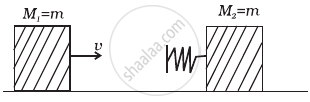1. While spring is fully compressed all the KE of M1 is stored as PE of spring.
2. While spring is fully compressed the system momentum is not conserved, though final momentum is equal to initial momentum.
3. If spring is massless, the final state of the M1 is state of rest.
4. If the surface on which blocks are moving has friction, then collision cannot be elastic.

#### VSA

Exercises | Q 6.22 | Page 45

A rough inclined plane is placed on a cart moving with a constant velocity u on horizontal ground. A block of mass M rests on the incline. Is any work done by force of friction between the block and incline? Is there then a dissipation of energy?

Exercises | Q 6.23 | Page 45

Why is electrical power required at all when the elevator is descending? Why should there be a limit on the number of passengers in this case?

Exercises | Q 6.24 (a) | Page 45

A body is being raised to a height h from the surface of earth. What is the sign of work done by applied force?

Exercises | Q 6.24 (b) | Page 45

A body is being raised to a height h from the surface of earth. What is the sign of work done by gravitational force?

Exercises | Q 6.25 | Page 45

Calculate the work done by a car against gravity in moving along a straight horizontal road. The mass of the car is 400 kg and the distance moved is 2 m.

Exercises | Q 6.26 | Page 45

A body falls towards earth in air. Will its total mechanical energy be conserved during the fall? Justify.

Exercises | Q 6.27 | Page 45

A body is moved along a closed loop. Is the work done in moving the body necessarily zero? If not, state the condition under which work done over a closed path is always zero.

Exercises | Q 6.28 | Page 45

In an elastic collision of two billiard balls, which of the following quantities remain conserved during the short time of collision of the balls (i.e., when they are in contact).

1. Kinetic energy.
2. Total linear momentum?

Exercises | Q 6.29 | Page 45

Calculate the power of a crane in watts, which lifts a mass of 100 kg to a height of 10 m in 20s.

Exercises | Q 6.30 | Page 46

The average work done by a human heart while it beats once is 0.5 J. Calculate the power used by heart if it beats 72 times in a minute.

Exercises | Q 6.31 | Page 46

Give example of a situation in which an applied force does not result in a change in kinetic energy.

Exercises | Q 6.32 | Page 46

Two bodies of unequal mass are moving in the same direction with equal kinetic energy. The two bodies are brought to rest by applying retarding force of same magnitude. How would the distance moved by them before coming to rest compare?

Exercises | Q 6.33 | Page 46

A bob of mass m suspended by a light string of length L is whirled into a vertical circle as shown in figure. What will be the trajectory of the particle if the string is cut at

1. Point B?
2. Point C?
3. Point X?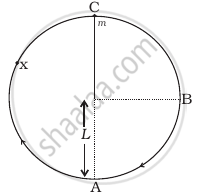#### SA

Exercises | Q 6.34 | Page 46

A graph of potential energy V(x) verses x is shown in figure. A particle of energy E0 is executing motion in it. Draw graph of velocity and kinetic energy versus x for one complete cycle AFA.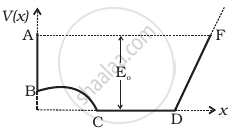Exercises | Q 6.35 (a) | Page 46

A ball of mass m, moving with a speed 2v0, collides inelastically (e > 0) with an identical ball at rest. Show that for head-on collision, both the balls move forward.

Exercises | Q 6.35 (b) | Page 46

A ball of mass m, moving with a speed 2v0, collides inelastically (e > 0) with an identical ball at rest. Show that for a general collision, the angle between the two velocities of scattered balls is less than 90°.

Exercises | Q 6.36 | Page 46

Consider a one-dimensional motion of a particle with total energy E. There are four regions A, B, C and D in which the relation between potential energy V, kinetic energy (K) and total energy E is as given below:

Region A : V > E
Region B : V < E
Region C : K > E
Region D : V > K

State with reason in each case whether a particle can be found in the given region or not.

Exercises | Q 6.37 | Page 47

The bob A of a pendulum released from horizontal to the vertical hits another bob B of the same mass at rest on a table as shown in figure.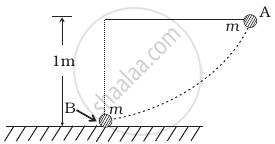If the length of the pendulum is 1 m, calculate

1. the height to which bob A will rise after collision.
2. the speed with which bob B starts moving. Neglect the size of the bobs and assume the collision to be elastic.
Exercises | Q 6.38 | Page 47

A raindrop of mass 1.00 g falling from a height of 1 km hits the ground with a speed of 50 ms–1. Calculate

1. the loss of P.E. of the drop.
2. the gain in K.E. of the drop.
3. Is the gain in K.E. equal to a loss of P.E.? If not why.

Take g = 10 ms–2

Exercises | Q 6.39 | Page 47

Two pendulums with identical bobs and lengths are suspended from a common support such that in rest position the two bobs are in contact (Figure). One of the bobs is released after being displaced by 10° so that it collides elastically head-on with the other bob.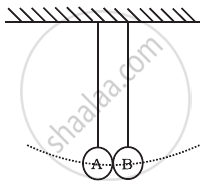1. Describe the motion of two bobs.
2. Draw a graph showing variation in energy of either pendulum with time, for 0 ≤ t ≤ 2T, where T is the period of each pendulum.
Exercises | Q 6.40 | Page 47

Suppose the average mass of raindrops is 3.0 × 10–5 kg and their average terminal velocity 9 ms–1. Calculate the energy transferred by rain to each square metre of the surface at a place which receives 100 cm of rain in a year.

Exercises | Q 6.41 | Page 47

An engine is attached to a wagon through a shock absorber of length 1.5 m. The system with a total mass of 50,000 kg is moving with a speed of 36 km h–1 when the brakes are applied to bring it to rest. In the process of the system being brought to rest, the spring of the shock absorber gets compressed by 1.0 m. If 90% of energy of the wagon is lost due to friction, calculate the spring constant.

Exercises | Q 6.42 | Page 47

An adult weighing 600 N raises the centre of gravity of his body by 0.25 m while taking each step of 1 m length in jogging. If he jogs for 6 km, calculate the energy utilised by him in jogging assuming that there is no energy loss due to friction of ground and air. Assuming that the body of the adult is capable of converting 10% of energy intake in the form of food, calculate the energy equivalents of food that would be required to compensate energy utilised for jogging.

Exercises | Q 6.43 | Page 48

On complete combustion a litre of petrol gives off heat equivalent to 3 × 107 J. In a test drive a car weighing 1200 kg. including the mass of driver, runs 15 km per litre while moving with a uniform speed on a straight track. Assuming that friction offered by the road surface and air to be uniform, calculate the force of friction acting on the car during the test drive, if the efficiency of the car engine were 0.5.

#### LA

Exercises | Q 6.44 | Page 48

A block of mass 1 kg is pushed up a surface inclined to horizontal at an angle of 30° by a force of 10 N parallel to the inclined surface (Figure). The coefficient of friction between block and the incline is 0.1. If the block is pushed up by 10 m along the incline, calulate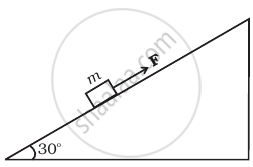1. work done against gravity
2. work done against force of friction
3. increase in potential energy
4. increase in kinetic energy
5. work done by applied force.
Exercises | Q 6.45 | Page 48

A curved surface is shown in figure. The portion BCD is free of friction. There are three spherical balls of identical radii and masses. Balls are released from rest one by one from A which is at a slightly greater height than C.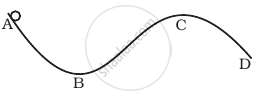With the surface AB, ball 1 has large enough friction to cause rolling down without slipping; ball 2 has a small friction and ball 3 has a negligible friction.

1. For which balls is total mechanical energy conserved?
2. Which ball (s) can reach D?
3. For balls which do not reach D, which of the balls can reach back A?
Exercises | Q 6.46 | Page 49

A rocket accelerates straight up by ejecting gas downwards. In a small time interval ∆t, it ejects a gas of mass ∆m at a relative speed u. Calculate KE of the entire system at t + ∆t and t and show that the device that ejects gas does work = (1/2)∆m u^2 in this time interval (neglect gravity).

Exercises | Q 6.47 | Page 49

Two identical steel cubes (masses 50 g, side 1 cm) collide head-on face to face with a speed of 10 cm/s each. Find the maximum compression of each. Young’s modulus for steel = Y = 2 × 1011 N/m2.

Exercises | Q 6.48 | Page 49

A baloon filled with helium rises against gravity increasing its potential energy. The speed of the baloon also increases as it rises. How do you reconcile this with the law of conservation of mechanical energy? You can neglect viscous drag of air and assume that density of air is constant.

## Solutions for Chapter 6: Work, Energy and Power

Exercises## NCERT Exemplar solutions for Class 11 Physics chapter 6 - Work, Energy and Power

Shaalaa.com has the CBSE Mathematics Class 11 Physics CBSE solutions in a manner that help students grasp basic concepts better and faster. The detailed, step-by-step solutions will help you understand the concepts better and clarify any confusion. NCERT Exemplar solutions for Mathematics Class 11 Physics CBSE 6 (Work, Energy and Power) include all questions with answers and detailed explanations. This will clear students' doubts about questions and improve their application skills while preparing for board exams.

Further, we at Shaalaa.com provide such solutions so students can prepare for written exams. NCERT Exemplar textbook solutions can be a core help for self-study and provide excellent self-help guidance for students.

Concepts covered in Class 11 Physics chapter 6 Work, Energy and Power are Introduction of Work, Energy and Power, Notions of Work and Kinetic Energy: the Work-energy Theorem, Kinetic Energy, Work Done by a Constant Force and a Variable Force, The Concept of Potential Energy, Conservation of Mechanical Energy, Potential Energy of a Spring, Various Forms of Energy : the Law of Conservation of Energy, Non - Conservative Forces - Motion in a Vertical Circle, Concept of Work, Collisions, Power.

Using NCERT Exemplar Class 11 Physics solutions Work, Energy and Power exercise by students is an easy way to prepare for the exams, as they involve solutions arranged chapter-wise and also page-wise. The questions involved in NCERT Exemplar Solutions are essential questions that can be asked in the final exam. Maximum CBSE Class 11 Physics students prefer NCERT Exemplar Textbook Solutions to score more in exams.

Get the free view of Chapter 6, Work, Energy and Power Class 11 Physics additional questions for Mathematics Class 11 Physics CBSE, and you can use Shaalaa.com to keep it handy for your exam preparation.